Latest Banking jobs   »

# Reasoning Ability Quiz For NABARD Grade A 2022- 31st August

Directions (1-5): Study the following information carefully and answer the questions given below.
Eight people are sitting in two parallel rows such that A, S, D and F are sitting in row 1 and facing North and Z, C, V and B are sitting in row 2 and facing South. Each row consists of five seats. Therefore, in the given arrangement each seat in a row faces another seat of the other row and the vacant seat of row 1 faces the vacant seat in row 2 and vice versa. D faces the one who sits to the immediate left of the one who faces A who is the neighbour of D. One person sits between D and F. B faces the one who sits second to the right of F. V faces the one who sits diagonally opposite of C but C does not face the one who is an immediate neighbour of D. Z sits to the immediate right of V but does not face F.

Q1. Who among the following sit to the immediate left of vacant seats in both rows?
(a) F, V
(b) F, B
(c) A, Z
(d) C, D
(e) None of the above

Q2. Four among the following five are alike in a certain way and hence form a group. Who among the following does not belong to the group?
(a) C
(b) F
(c) S
(d) V
(e) D

Q3. Which of the following statement is not true?
(a) V faces S
(b) Z sits to the immediate left of the one who faces A
(c) One person sits between B and V
(d) One person sits between D and S
(e) None of the above

Q4. What is the position of B with respect to the one who faces S?
(a) Immediate right
(b) Immediate left
(c) Second to the right
(d) Second to the left
(e) None of the above

Q5. If F is related to C, in the similar way B is related to A, then who among the following is related to Z?
(a) F
(b) A
(c) D
(d) S
(e) B

Directions (6-10): Study the following information carefully and answer the questions given below.
Few persons were sitting around a circular table facing away from the centre. The seats were placed at an equal distance. The number of persons around the circular table was neither more than 21 nor in the multiple of 5. S is to the immediate right of N. Q was second to the left of Z and second to the right of E, who was seated to the immediate right of Y. There were three seats between Y and V. S was third to the left of V. Number of persons between P and S when counted from the left of P was one third of the number of persons between P and N when counted from the right of P.

Q6. How many persons were seated around the table?
(a) 20
(b) 19
(c) 15
(d) 21
(e) None of these

Q7. What is the position of E with respect to P?
(a) Third to the left
(b) Fourth to the right
(c) Third to the right
(d) Fourth to the left
(e) None of these

Q8. How many persons were seated between N and Z?
(a) One
(b) Four
(c) Six
(d) Seven
(e) None of these

Q9. Which of the following statements is true?
(a) V is the immediate neighbour of P
(b) P sits second to the left of V
(c) There is only one seat between Y and P when counted from the right of P
(d) The seats between Q and P are more than 4, when counted from the right of P
(e) None is true

Q10. Who among the following sits fifth to the right of Y?
(a) N
(b) P
(c) Z
(d) S

Directions (11-13): Study the following information carefully and answer the questions given below.
Point X is 30m east of point Y and 20m north of point W. Point T is 10m north of point S and point U is 20m east of point T. Point Q is 20m west of point P. Point M is 30m north of point N and 40m west of point O. Point V is 40m north of point U and 30m east of point W. Point R is 30m south of point Q and 40m west of point S. Point P is 20m south of point O.

Q11. Point Y is in which direction of point Q?
(a) West
(b) South
(c) North
(d) South-West
(e) None of these

Q12. What is the shortest distance between M and W?
(a) 60m
(b) 70m
(c) 50m
(d) 40m
(e) Can’t be determined

Q13. Point T is in which direction of point Q?
(a) South-East
(b) North-West
(c) North-East
(d) South-West
(e) Can’t be determined

Directions (14-15): Study the following information carefully and answer the questions given below.
Point A is 6m to the west of point B. Point D is 4m to the west of point C. Point G is 9 to the south of point B. Point C is 5m to the north of point G. Point E is 6m to the west of point F. Point D is 5m to the north of point E.

Q14. What is the distance between point C and point B?
(a) 5m
(b) 4m
(c) 3m
(d) 2m
(e) 6m

Q15. If a person is standing at point A he walks towards his south and walks for 4m then he takes a left turn and walks for 8m and stopped at point K then point K is in which of the following direction with respect to point G?
(a) North-west
(b) South-west
(c) North-east
(d) South-east
(e) South

Solutions

Solution (1-5):
Sol.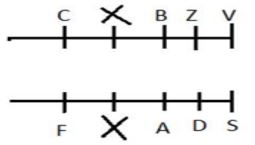S1. Ans. (b)
S2. Ans. (e)
S3. Ans. (d)
S4. Ans. (c)
S5. Ans. (c)

Solution (6-10):
Sol.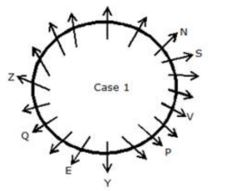S6. Ans. (b)
S7. Ans. (c)
S8. Ans. (e)
S9. Ans. (c)
S10. Ans. (c)

Solution (11-13):
Sol.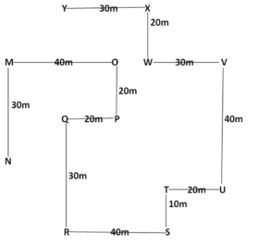S11. Ans. (c)
S12. Ans. (c)
S13. Ans. (a)

Solution (14-15):
Sol.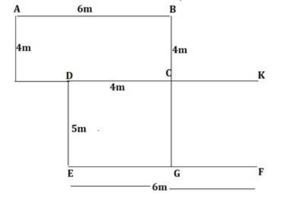S14. Ans. (b)
S15. Ans. (c)#### Congratulations!Download Hindu Review of October 2021: Free PDFIncorrect details? Fill the form again here

Download Hindu Review of October 2021: Free PDF

Thank You, Your details have been submitted we will get back to you.
•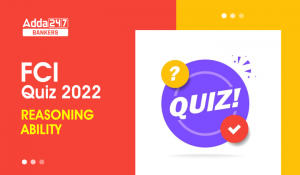Reasoning Ability Quiz For FCI Phase I 2...
•Education Minister Dharmendra Pradhan in...
•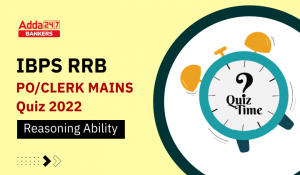Reasoning Quizzes For IBPS RRB PO/Clerk ...
•BSE receives SEBI's final approval to la...
•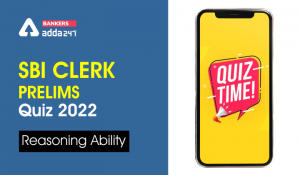Reasoning Ability Quiz For SBI Clerk Pre...
•World Tourism Day 2022, Theme, History &...